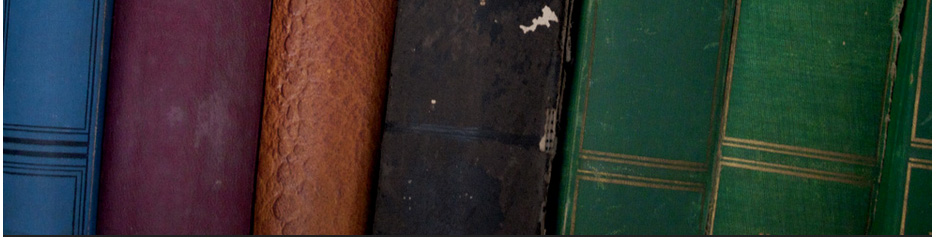## Secondary education# MATHS KS3 : Equations [solving linear equation]

ks3mat0001 An introduction to equations

ks3mat0002Solving linear equations easily

ks3mat0003 Equations with fractions - Higher - Test

ks3mat0004 Equations with variables on both sides

ks3mat0006 Solving equations with one unknown

ks3mat0008Solving Equations in One Variable

ks3mat0009 Solving_Linear_Equations

ks3mat0010 Multi-Step Equations

ks3mat0011 Solving linear equations

Ermias Home

HAREP The Horn of Africa Research and Knowledge Exchange Platform © 2010-2012

 Contact: rkidane@talk21.com Please click Here to send your feedback